### Home > PC > Chapter 8 > Lesson 8.2.5 > Problem8-139

8-139.

1. Apply the “Fibonacci rule” to these two beginning numbers. Then compute aj for j = 3, 4, …, 10, 11.

2. Find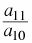(round off to the nearest 0.001).

3. Find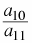(round off to the nearest 0.001).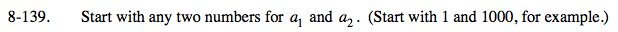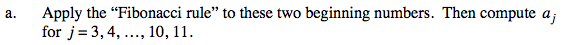a1 = 1
a2 = 1000
a3 = 1 + 1000 = 1001
a4 = 1000 + 1001 = 2001
a5 = 1001+ 2001 = 3002
a6 = 2001+ 3002 = 5003
a7 = 3002 + 5003 = 8005
a8 = 5003 + 8005 = 13008
a9 = 8005 + 13008 = 21, 013
a10 = 13008 + 21013 = 34, 021
a11 = 21013 + 34021 = 55, 034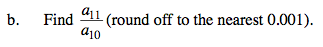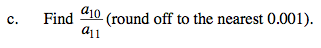0.618